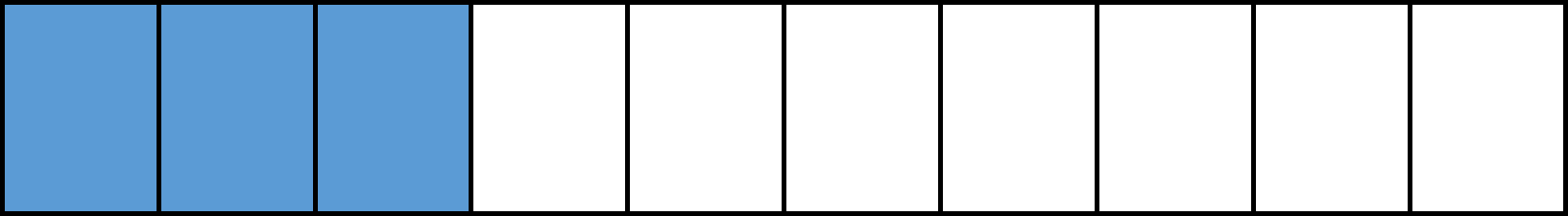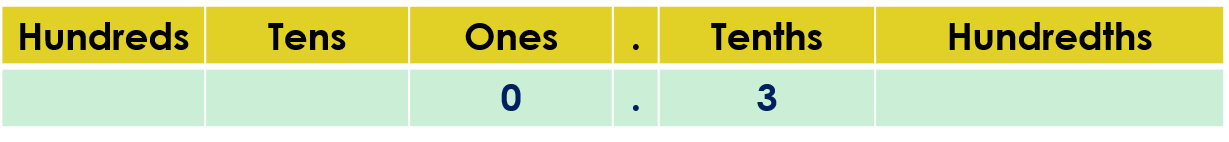1
visibility

What decimal number is represented?• A

0.13

• B

0.03

• C

0.3

• D

0.1

The correct answer is "0.3."

Solution:

Given the model below:We observe that 3 out of  10 parts are colored.

That is, the part shaded in the model is three tenths.

We write three tenths as a decimal.Three tenths is written as the decimal 0.3.​

So, 0.3 represents the given model.